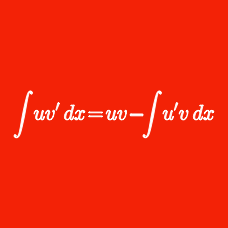Calculus

# Integration U-substitution - Trigonometric

Evaluate the integral $$\displaystyle{ \int { \frac{10x}{\sqrt{3-2x-x^2}} dx }} ,$$ using $$C$$ as the constant of integration.

Evaluate the integral $$\displaystyle{ \int { \frac { 47 \sqrt{9-x^2} }{x^2 } dx } },$$ using $$C$$ as the constant of integration.

Evaluate the integral $$\displaystyle{ \int{\frac{15}{x^2\sqrt{x^2+4}}dx}},$$ using $$C$$ as the constant of integration.

Evaluate the integral $$\displaystyle{ 43\int { \cos^3 x dx} },$$ using $$C$$ as the constant of integration.

Given $$a>0 ,$$ evaluate the integral $$\displaystyle{ 41 \int { \frac{dx}{\sqrt{x^2 - a^2}} }},$$ using $$C$$ as the constant of integration.

×

Problem Loading...

Note Loading...

Set Loading...